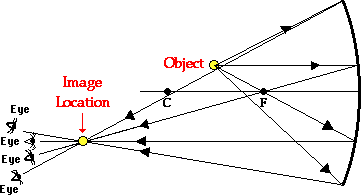Home » Posts tagged 'optics'

# Tag Archives: optics

### Subscribe to this site by email## BOARD QUESTIONS FROM WAVE OPTICS (CBSE/AISSCE)

Practising the previously asked questions is a good idea to prepare for the exams.

## Optics

“when we exchange the lenses of compound microscope, then why it does not act as a telescope?”

## How to walk through walls?

Illusion optics create an invisible gateway

Chinese researchers have devised an invisible gateway.## Optics MCQ – image formation by concave mirror

I am having trouble with a problem.

Q. In an experiment to determine the focal length (f) of a concave mirror by the u–v method, a student places the object pin A on the principal axis at a distance x from the pole P. The student looks at the pin and its inverted image from a
distance keeping his/her eye in line with PA. When the student shifts his/her eye towards left, the image appears to the right of the object pin. Then

(A) x < f

(B) f < x < 2f

(C) x = 2f

(D) x > 2fEXPLANATION

The image and the object will move together at 2f

The one closer to the eye will move faster

In the question, the situation occurs when the image is closer to the eye and this happens when the object is between f and 2f

## A Question from Optics (awaiting answer)

Question: The difference between the speed of light compared to any other body on earth is negligible then if we take a car moving at 30m/s and i stand at a distance of 1m from it i am a stationary observer,if i assume the speed of light to be 3 x 10^8 then even i will observe the world at such a speed then when i look at the car say for one second then light has already reached my eye after bouncing off the car, by that time the car would have moved a very very small distance and this repeats until the car moves past me.what i cant understand is then why does the car whizz past me because at that rate it should take a lot of time to go past me and i should be able to see the cars every nanometer of motion just like in slow motion videos.i have read in a psychology book that it is because of the angle made by the retina of the eye and the objectively regardless of my human perception what could be the reason?

## A numerical Problem from optics

When an object is kept at a distance of 60cm from a concave mirror, the magnification is 1/2. Where should the object be placed to get magnification of 1/3??

Ans:

In the first part, u=-60, m=(-)1/2 implies v=-30 cm (Since the image must be real as concave lens form virtual image of bigger size only)

we get f = –20 cm

In II case

m=-1/3

v=u/3

f=-20cm

we get u=4f=4 x (-20)=-80 cm

## Question from Wave Optics

What is the difference between interference and diffraction?

Ans:

Interference is caused by the superposition of wave fronts from two coherent sources; But diffraction is caused by the superposition of wavelets from a single wavefront when it confronts an obstacle or a slit.

### Hits so far @ AskPhysics

• 2,240,387 hits